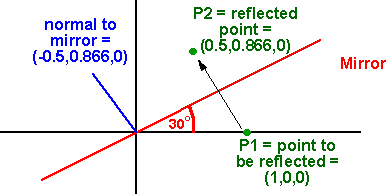# Maths - Reflection using Matrix

### Matrix representation

#### normal component

Norm = VaP = (Va × P) * P/|P|²

#### parallel component

Proj = Va || P = Va • P * P/|P|²

#### Reflection matrix

Given the inversion I'll add the terms instead of subtracting them to give the reflection result:

p -> 1 /
(Px² + Py² + Pz²)*
 -Px² + Pz²+ Py² - 2 * Px * Py - 2 * Px * Pz - 2 * Py * Px -Py² + Px² + Pz² - 2 * Py * Pz - 2 * Pz * Px -2 * Pz * Py -Pz² + Py² + Px²
[p]

Note that this matrix is symmetrical about the leading diagonal, unlike the rotation matrix, which is the sum of a symmetric and skew symmetric part.

#### Simple cases

In order to check the above lets take the simple cases where the point is reflected in the various axis:

Reflection in yz

 -1 0 0 0 1 0 0 0 1

Reflection in xz

 1 0 0 0 -1 0 0 0 1

Reflection in xy

 1 0 0 0 1 0 0 0 -1

#### Determinant and eigen values

Another check is that the determinant of reflection matrix is -1

#### ExampleAs an example we want to reflect the point (1,0,0) in a plane at 30 degrees.

P2 =
 -Px² + Pz² + Py² - 2 * Px * Py - 2 * Px * Pz - 2 * Py * Px -Py² + Px² + Pz² - 2 * Py * Pz - 2 * Pz * Px -2 * Pz * Py -Pz² + Py² + Px²
[P1]

where Px,Py,Pz is the normal to the mirror which is: (-0.5,0.866,0)

and P1 is the initial point which is (1,0,0)

substituting these values gives:

P2 =
 -0.25 + 0 + 0.75 - 2 * -0.433 0 - 2 * -0.433 -0.75 + 0.25 + 0 0 0 0 0 + 0.75 + 0.25

 1 0 0

P2 =
 0.5 0.866 0 0.866 -0.5 0 0 0 1

 1 0 0

multiplying to vector by the matrix gives:

P2 =

 0.5 0.866 0Clifford Algebra to Geometric Calculus: A Unified Language for Mathematics and Physics (Fundamental Theories of Physics). This book is intended for mathematicians and physicists rather than programmers, it is very theoretical. It covers the algebra and calculus of multivectors of any dimension and is not specific to 3D modelling.